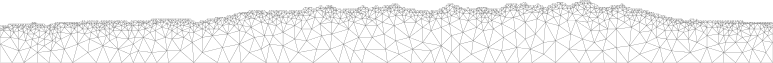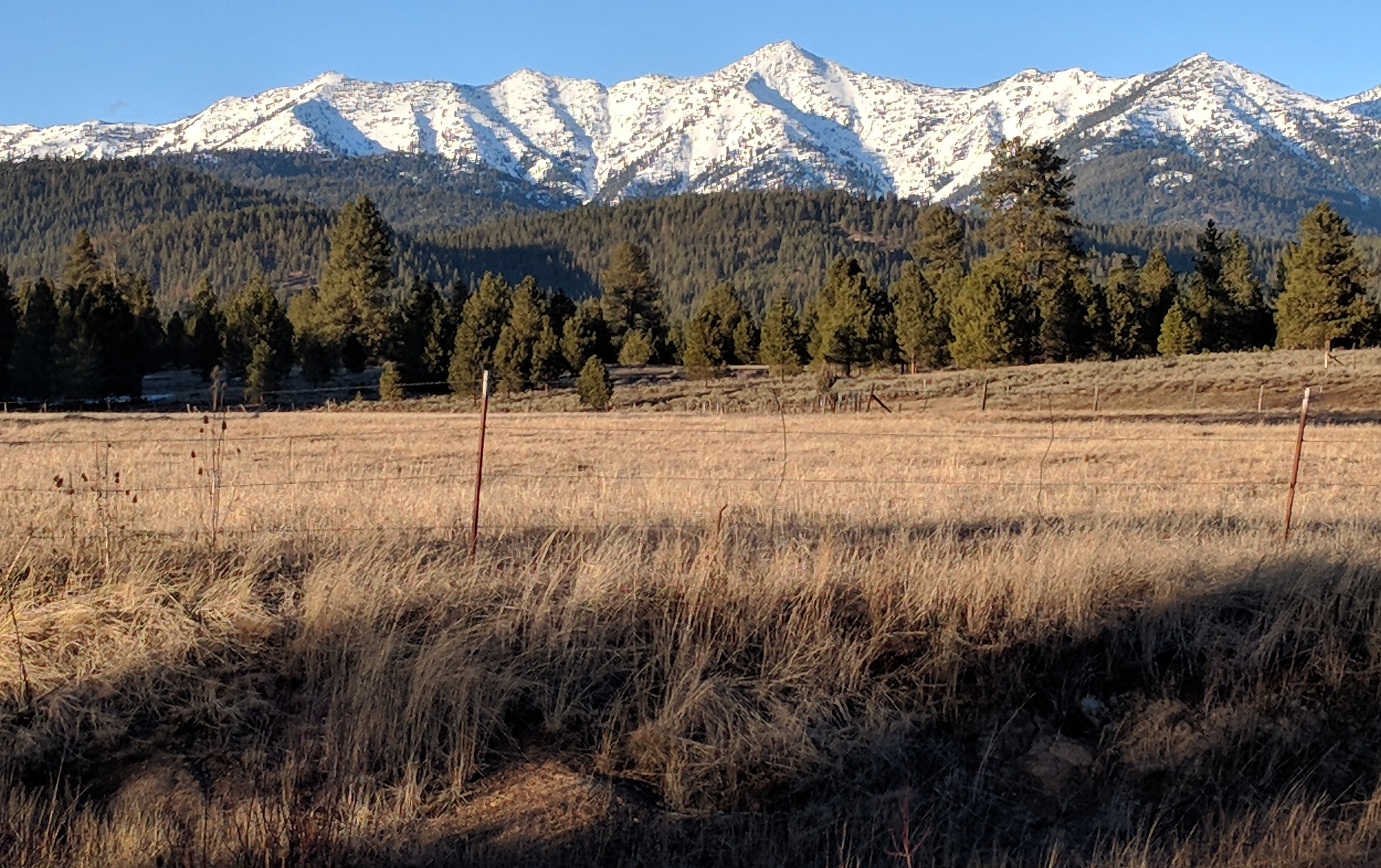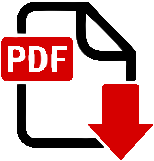Lesson Plan: Math for Geos | Jonathan Perry-HoutsJonathan Perry-Houts

### Lesson Plan: Math for GeosAs part of my Graduate Teaching Initiative advanced certificate, I developed a lesson for the Earth Sciences 202 course to introduce some basic mathematical concepts in the intro series curriculum. As a GTI project, I formulated this lesson as a small experiment, to test different techniques for introducing math to geology majors at the introductory level. You can read the full text of my proposal with justification for each pedagogical choice here. Feel free to contact me directly for answer keys to the assignments.

Goal: Improve students' confidence translating between math and native language.

Objective: Increase fluency in mathematics. Practice identifying important variables, identifying where mathematical constructs can describe nature.

Outcomes: Students will be able to formulate a basic differential equation that describes a natural system.

The module includes a homework assignment, a lecture with active learning exercises, a lab assignment, and some final exam questions. The full sequence of components, as I assigned it, is as follows:

### Homework

Students receive the homework a week before lecture is scheduled, to be due in class on the day of the lecture.

There are two versions of the same homework assignment, split evenly among the students. Some students get an assignment with rote problem solving exercises, designed to illustrate possible applications of math in geosciences. The other students get an assignment with conceptual questions, designed to encourage student to identify applications of math in geosciences. In lab, the two groups of students will interact in a sort of jig-saw-style activity.

### Lecture

Lecture is an hour and 20 minute slot.

The time is broken up by one short think-pair-share, and one longer worksheet assignment. The lecture time is structured to give a brief introduction, followed by a short lecture on the social history of calculus, and an interactive lecture on what derivatives mean in physical terms.

There is time in the lecture devoted to talking about stereotype threat and diversity in STEM, following the conclusions of  that discussing stereotype threat improves outcomes for students of underrepresented groups. As an aside, I was skeptical that just mentioning something could make a difference in such a complex problem, but I actually got feedback from a few students who said that short bit of lecture made a difference for them.

During lecture I show the students the exact question that will be on their final exams, giving them about three weeks to work on their answers.

### Lab

The lab activity is a mix of questions that students with the different homework versions will be differently prepared for. In principle, students who did the conceptual homework should be well practiced at conceptual questions, and vice versa. Students work in heterogeneous groups of up to three students, with at least one member who did each homework version. The intent of this structure was to encourage a jig-saw like collaboration among students from the two groups.Lab Worksheet

### Final Exam

The final exam includes one take-home question based on this module. The question is shown during lecture, and students are encouraged to copy it down. It is designed to test high level comprehension of the module objectives. The question reads:

Consider the statement:
"The rate at which coffee loses heat is proportional to how hot it is."

b) Describe a different system (not temperature-related) that obeys the same
type of equation. Explain your reasoning.


There are also several multiple-choice questions to test students ability to recognize mathematical constructs in physical systems.

### Reflections

I ran this in two lab sections, one in which students collaborated with others who did the same homework version, and one where they collaborated across homework versions. My goal was to test for correlations between an individual's lab group, and their performance on the lab activity or the final exam. The small sample size of the course didn't give conclusive results one way or another, but my impression was that this homework/lab structure was more confusing than its outcome justified.

In the future I think this format should probably be modified to combine the homework questions into one shared assignment, and run the homework/labs in a more traditional format. The concept of testing different exercise formats is something I'm interested in pursuing, but will need to be implemented as a longer-term project with stronger experimental controls.

 Johns, Michael, Toni Schmader, and Andy Martens. "Knowing is half the battle: Teaching stereotype threat as a means of improving women's math performance." Psychological Science 16.3 (2005): 175-179. https://doi.org/10.1111/j.0956-7976.2005.00799.x

Written on August 20th, 2018 by JPH